Journal of Vibroengineering

Published: 25 August 2022

# Study on vibration characteristics of ship mining system under composite load

Xiao Shi1
Dan Li2
Aixia Zhang3
Jian Zhang4
1, 2, 3, 4Qingdao Huanghai University, Qingdao, China
Corresponding Author:
Jian Zhang
Views 38

#### Abstract

The load that borne by ship mining system is very complex. The vibration of lifting pipe can be significantly affected by ocean current and wind load, which has a key impact on material lifting, ore bin storage and ship towing. Considering the composite load condition, the vibration control equation of mining ship is established based on the transverse swing mechanism of ship hull, and the variation law of transverse disturbance is obtained. The dynamic equation is constructed according to the bearing characteristics of the lifting system and the D’Alembert principle. The Wilson method is used to analyze and obtain the transverse vibration spectrum response of the lifting pipe under different wind loads, hull disturbance velocity and water depth. Based on the analogy method and hammering method, the vibration feedback test-bed of the lifting system is built, and the time-domain and frequency-domain vibration characteristics of the lifting pipe model under different water depths are obtained. The results show that in shallow water, the disturbance of mining ship and the composite load of ocean current are the key factors affecting the vibration amplitude of lifting pipe respectively. With the increase of water depth, the characteristic frequency and amplitude of the system decrease, and the amplitude gradually presents a discontinuous phenomenon.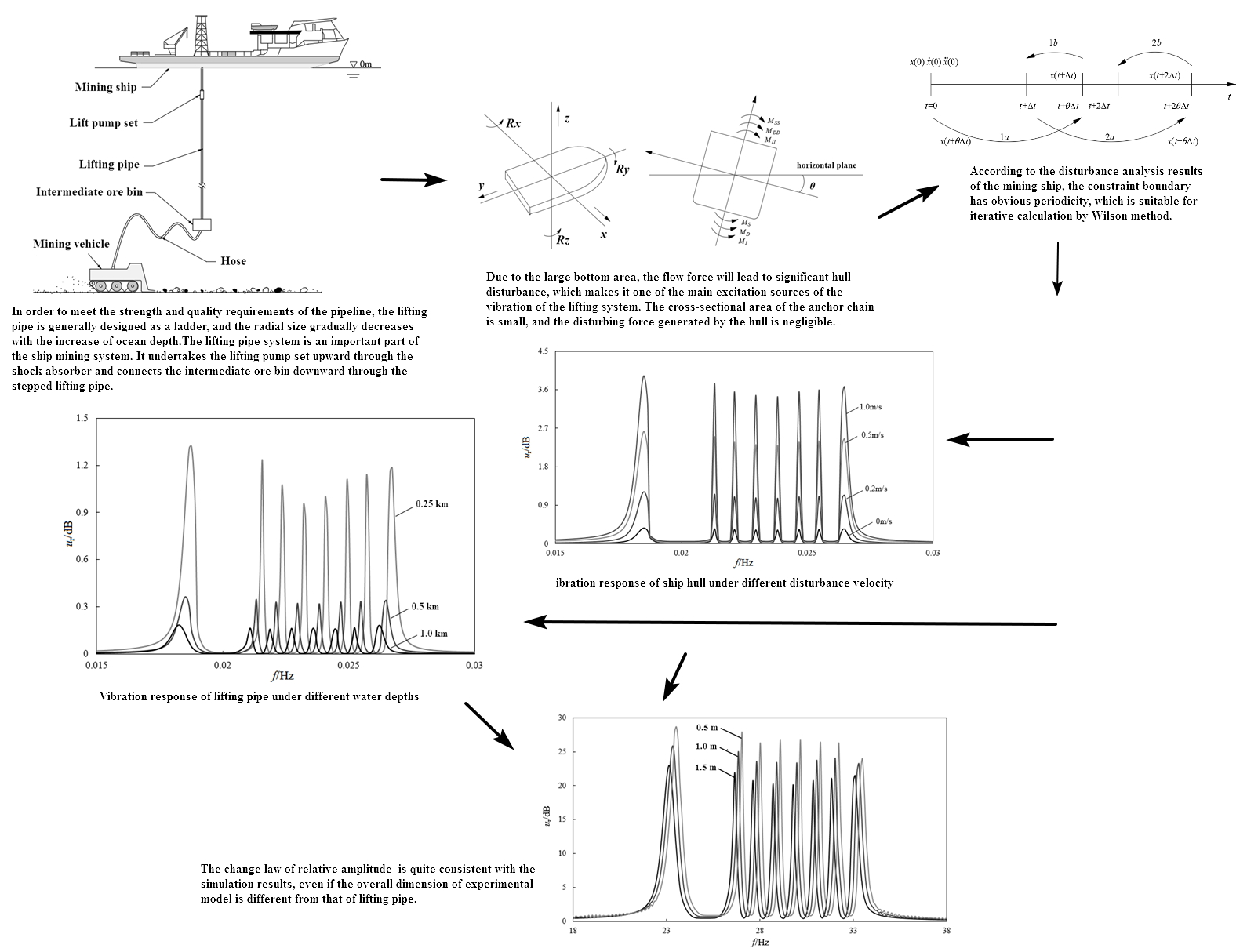#### Highlights

• Considering the composite load condition, the vibration control equation of mining ship was established based on the transverse swing mechanism of ship hull.
• The Wilson method is used to analyze and obtain the transverse vibration spectrum response of the lifting pipe under different wind loads, hull disturbance velocity and water depth.
• Based on the analogy method and hammering method, the vibration feedback test-bed of the lifting system was built.

## 1. Introduction

The ocean is rich in mineral resources. Mining in the deep sea is the destination and direction of energy development in the future. The development of seabed mining technology is not only the demand of national strategy , but also one of the most effective ways to make up for the shortage of land resources. In recent years, with the development of science and technology, the research on the field of deep-sea mining has received strong support from various countries. As the important seabed mining scheme, ship mining has a water depth of more than 5 km in the field of mineral exploration and development. The relevant research methods have also reached the leading level of technology and achieved good application results. At present, representative research achievements are as follows: Song  established a dynamic model with linkage characteristics according to the motion law of seabed mining vehicle, which can verify the safety and feasibility of tensioners and other components; Xu  used SESAM software to establish the coupling analysis model of mining ship and slurry lifting pipeline, and studied the fatigue damage characteristics of lifting pipeline; Chen  used the concentrated mass method to analyze the load characteristics of the lifting pipeline and numerically simulated the stress characteristics of the end shaft section; Ding  established a two-phase flow finite element simulation model to analyze the flow state of solid-liquid mixed materials in the lifting pipe; Song  studied the nonlinear transient equation of the stepped lifting pipe, and mainly analyzed the variation law of the axial stress of the pipe with time; Rashidov  simplified the lifting pipe into a beam element finite element model, compiled a nonlinear static analysis program, and analyzed the equilibrium shape and stress state of the lifting system under the given boundary conditions; Slam  proposed to use the discrete element method based on multi rigid body dynamics to calculate and analyze the vibration of the ore lifting pipe, and compared with the early finite element calculation results.

However, the current research is still insufficient for the research of composite load, and it is difficult to obtain reliable vibration response results. Lifting pipeline is the key equipment for long-distance material transportation. Under the combined action of mining ship and ocean current, it presents significant and complex nonlinear vibration characteristics . Local strong vibration will not only produce large pipe deflection, induce strength damage and fatigue damage , but also inhibit the mobility and flexibility of shipping equipment. The vibration characteristic of lifting pipe system is an important basis for the reliability design and optimization design of ship mining system. It is necessary and urgent to study the vibration response of lifting pipe under different working conditions, especially composite loads, like wind load and ocean current load.

## 2. Bearing characteristics of ship mining system

### 2.1. Overall system structure

The mainstream ship mining system is mainly composed of mining ship, pipe lifting system, ore collection system and mining vehicle. Its cooperative working principle is shown in Fig. 1. This type of mining system has high flexibility and integration, high safety, scattered power sources , convenient equipment maintenance and good business prospects. However, during operation, due to the large length of the ore lifting pipe, vibration is easily produced under the action of ocean current, which brings great inconvenience to material lifting, ore bin storage and ship towing. In the whole deep-sea mining operation, the ore collection system is connected with the mining vehicle through a hose mechanism, and the ore is initially broken and decontaminated before entering the ore bin. In order to meet the strength and quality requirements of the pipeline, the lifting pipe is generally designed as a ladder, and the radial size gradually decreases with the increase of ocean depth.

Fig. 1Overall structure of ship mining system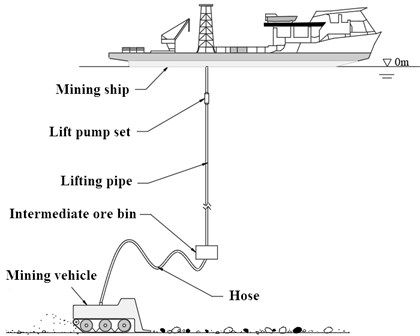### 2.2. Bearing analysis of lifting system

The lifting pipe system is an important part of the ship mining system. It undertakes the lifting pump set upward through the shock absorber and connects the intermediate ore bin downward through the stepped lifting pipe. The stress diagram of the lifting system is shown in Fig. 2. It can be seen that the action of ocean current is one of the key factors affecting the vibration of the lifting. Under the action of ocean current load $F\left(x\right)$, the node displacement response of lifting pipe is $u\left(t\right)$, and its radial and axial vibration components are ${u}_{1}\left(t\right)$ and ${u}_{2}\left(t\right)$ respectively.

In essence, ocean current refers to the large-scale transfer of seawater from one sea area to the other, which is a special current movement with obvious interaction effect with waves. According to the cause of formation , ocean current can be divided into wind current, density current and wave current, and nearshore current and offshore current according to different flow ranges. The speed and direction of ocean current movement is changing slowly with time and space. Therefore, in the actual ocean engineering calculation, ocean current is generally regarded as steady-state current . The velocity of ocean current is different at different ocean depths. Through the test and exponential function fitting, it can be concluded that the functional relationship between the water flow rate ${V}_{c}$ within 5 km of the vertical deep sea and the sea depth $z$ under the condition of four-level wind load  is:

1
${V}_{c}=0.1+1.6×{\left(\frac{5000+z}{5000}\right)}^{12}.$

Fig. 2Bearing diagram of lifting system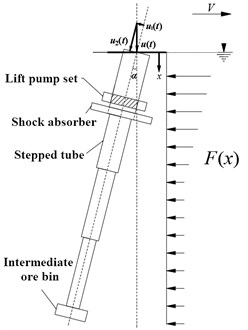The curve between ${V}_{c}$ and $z$ can be drawn by MATLAB, as shown in Fig. 3. It can be seen that when the ocean depth exceeds 2 km, the water flow rate hardly changes and remains at about 0.1 m/s. The flow rate in the whole shallow water area (water depth 0-1 km) is greatly affected by water depth, and the flow rate at sea level is the largest, which decreases sharply with the increase of water depth. In moderate waters (water depth 1-2 km), the influence of water depth on water flow rate gradually weakens. The ocean current in shallow water has the most significant force on the lifting pipe system. According to the test, under the action of different wind loads, the changes of hydrodynamic load per unit area of lifting pipe in water depth of 0-1 km show significant consistency with the changes of water flow rate.

Fig. 3Relationship between current velocity and sea depth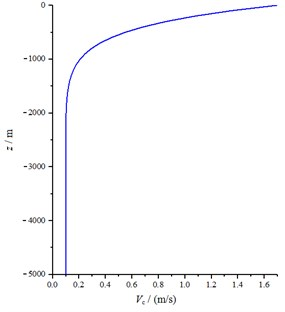### 2.3. Hull disturbance analysis

When the ship mining system works, the anchor chain can effectively restrict the displacement of the ship hull. However, due to the large bottom area, the flow force will lead to significant hull disturbance, which makes it one of the main excitation sources of the vibration of the lifting system. The cross-sectional area of the anchor chain is small, and the disturbing force generated by the hull is negligible. It is assumed that the center of gravity of the mining ship is located at the waterline, and the disturbance model is a six degree of freedom rigid body. In terms of vibration direction, set $x$ direction as transverse, $y$ direction as longitudinal and $z$ direction as vertical, as shown in Fig. 4. Taking the transverse plane vibration as the research object, set the active towing moment of the ocean current as ${M}_{S}$, the inertial towing moment as ${M}_{I}$, the damping towing moment as ${M}_{D}$, the active disturbance moment of the wave as ${M}_{SS}$, the inertial disturbance moment as ${M}_{II}$, the damping disturbance moment as ${M}_{DD}$, and the included angle between the hull and the horizontal plane as $\theta$. Then the governing equation of motion can be expressed as:

2
${M}_{S}+{M}_{D}+{M}_{I}={M}_{SS}+{M}_{DD}+{M}_{II},$
3
${M}_{S}={V}_{t}{h}_{a}\theta ,$

where ${V}_{t}$ is the displacement of mass the center, ${h}_{a}$ is the height of the transverse mass center.

The superposition of ocean current and wave determines the disturbance law of the hull, and the hull disturbance further affects the vibration of the lifting system. Based on the lateral sway mechanism and Newton's second law, the hull disturbance equation can be characterized as:

4
${V}_{t}{h}_{a}\theta +2{N}_{\theta }{\theta }^{\text{'}}+\left({I}_{xx}+{J}_{xx}\right){\theta }^{\text{'}\text{'}}={V}_{t}{h}_{a}{a}_{\theta }+2{N}_{\theta }{{a}_{\theta }}^{\text{'}}+{J}_{xx}{{a}_{\theta }}^{\text{'}{}^{\text{'}}}.$

where ${N}_{\theta }$ is the disturbance damping coefficient, ${I}_{xx}$ is the moment of inertia, ${J}_{xx}$ is the additional moment of inertia, ${a}_{\theta }$ is the inclination of the superimposed force.

Fig. 4Hull disturbance model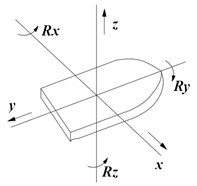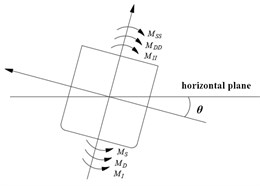Fig. 5Variation of transverse included angle between hull and horizontal plane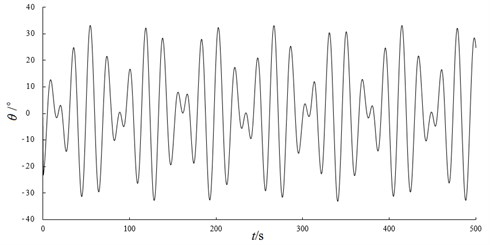Under severe wind load conditions (extreme working condition, level 8 transverse wind load), the disturbance law can be analyzed according to the structural parameters, draft state and disturbance equation of the mining ship, as shown in Fig. 5. It can be seen that the hull disturbance has obvious periodicity, but the characteristic frequency is very low. At a specific ocean current rate, it is easy to cause resonant excitation force to the lifting pipe and significantly amplify the amplitude of the pipe.

## 3. Influence of working conditions on lateral vibration

### 3.1. Dynamic equation

The load of the lifting pipe system is complex and changeable. In addition to the composite flow load, the restrained end is also affected by the hull disturbance, which makes the lifting pipe have significant forced vibration in both transverse and longitudinal directions. The influence of different loads and constraints on vibration is expressed in the form of amplitude superposition , which is easy to cause damage and failure of the whole system, such as material blockage, pipe burst, overload deformation, etc., and reduce the working life of the lifting pipe. There are many factors affecting the composite load, such as wind load, ore bin storage, hull disturbance speed, lifting pipe length and so on. In the dynamic equation , these contributing factors can be expressed in the form of stiffness matrix and damping matrix. According to the bearing response diagram shown in Fig. 2 and the D’Alembert principle, the dynamic equation of the lifting system can be expressed as:

5
$\left[M\right]u\text{'}\text{'}\left(t\right)+\left[C\right]u\text{'}\left(t\right)+\left[K\right]u\left(t\right)=F\left(t\right),$

where [$M$], [$C$] and [$K$] represent the stiffness, damping and mass matrix of the lifting system, $F\left(t\right)$ is the composite load matrix.

Due to the complexity and nonlinearity, it is difficult to get the dynamic equation of the lifting system by using the direct integration method. However, according to the disturbance analysis results of the mining ship, the constraint boundary has obvious periodicity, which is suitable for iterative calculation by Wilson method . The principle of this calculation method is shown in Fig. 6. Firstly, the overall stiffness, mass and damping matrix are determined. Then, given the initial value, select the time step and calculate the integral constant to meet the requirements of unconditional stability. Finally, the equivalent stiffness matrix is formed, and the parameters such as displacement, velocity and acceleration at the next moment are calculated iteratively. This research method can obtain higher calculation efficiency and good convergence effect. The kinematic parameters can be solved by setting virtual positioning points through links RT real-time simulation software.

Fig. 6Solving principle of Wilson method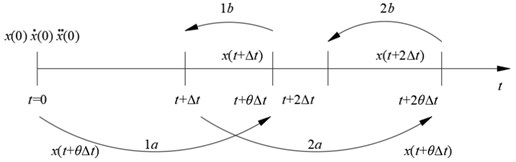### 3.2. Vibration characteristics under different wind loads

According to Eq. (1), the wind load is converted to the ocean current rate. Taking the minimum disturbance of the mining ship as the boundary, the relationship curve between the relative amplitude ${u}_{r}$ and the excitation frequency $f$ of the lifting pipe at the water depth of 500 m under different wind loads can be calculated, as shown in Fig. 7. The formula for calculating the relative amplitude ${u}_{r}$ is:

6
${u}_{r}=20\mathrm{l}\mathrm{g}\frac{{a}_{n}}{{a}_{0}},$

where ${a}_{n}$ is the transient amplitude, ${a}_{0}$ is the reference amplitude at time 0, so as to ensure that the initial relative amplitude of the spectral response is 0.

It can be seen from Fig. 7 that there are multiple characteristic frequencies in the range of 0.03 Hz for the lifting system, and the wind load has a certain impact on the characteristic frequency of the system. Under different resonance frequencies, the relative amplitude of specific wind load remains basically unchanged. At a specific resonance frequency, the relative amplitude under level 6 wind load is more than 50 % higher than that under level 4 wind load.

Fig. 7Vibration response of lifting pipe under different wind loads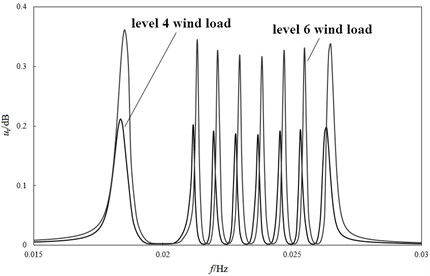### 3.3. Vibration characteristics of ship hull under different disturbance velocities

Hull disturbance is the result of the combined action of wind load and current. Under the condition of f level 4 wind load, the relationship curve between the relative amplitude and excitation frequency of the lifting pipe at the water depth of 500 m can be obtained, as shown in Fig. 8. It can be seen that the hull disturbance velocity has no effect on the characteristic frequency of the lifting pipe system, but the larger hull disturbance will cause the significant increase of the relative amplitude of the lifting pipe. Compared with wind load, the disturbance of mining ship has a more significant impact on the vibration of lifting system. When the ship mining system works, it is necessary to ensure that the mining ship is stably maintained in the effective resource area. However, there are often multiple mining areas in the whole project, and the installation and disassembly process of the lifting system is very cumbersome and time-consuming, which requires the mining ship to have great anti disturbance ability. It can be seen that strictly controlling the disturbance of mining ship through technical means is one of the most effective ways to reduce the vibration amplitude of lifting pipe, and it is also the key condition to ensure stable and efficient production.

Fig. 8Vibration response of ship hull under different disturbance velocity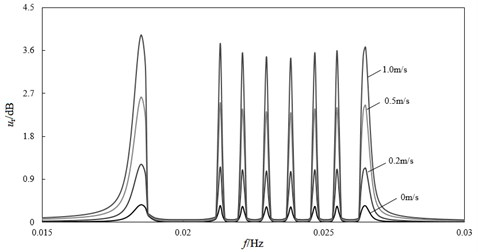### 3.4. Vibration characteristics of different water depths

According to the bearing characteristics of the lifting pipe system, the ocean current under different water depths has different effects on the lifting pipe. Considering the mass change of ore lifting pipe, the vibration response results of ore lifting pipe under different water depths in shallow water can be calculated, as shown in Fig. 9. It can be seen that the characteristic frequencies of lifting pipe nodes at different water depths are different. With the increase of water depth, the characteristic frequencies show a decreasing trend. When the water depth is 250 m, the peak amplitude decreases first and then increases with the increase of excitation frequency. The ocean current load in the deep sea is weak, so the relative amplitude of the lifting pipe at the depth of 1 km is small. However, due to the continuity of the lifting pipe structure and large axial size, the overall deflection affected by the disturbance of the top hull is more significantly.

Fig. 9Vibration response of lifting pipe under different water depths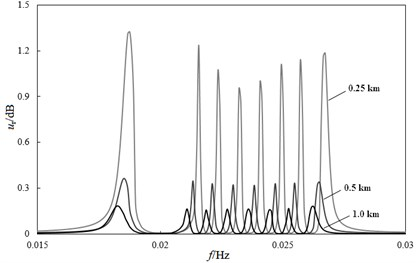## 4. Vibration test of lifting system

### 4.1. Design of test bench

In order to verify the vibration calculation results of the lifting system, according to the bearing characteristics of the lifting system, a vibration feedback test-bed of the lifting system is built based on the principles of analogy method and hammering method, as shown in Fig. 10.

Fig. 10Lifting system test bench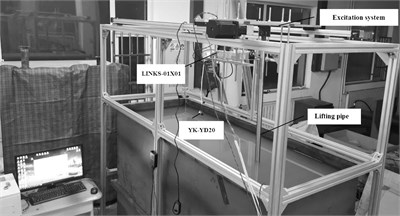In the test bench, the lifting pipe is a stainless steel pipe with a length of 2 m, an outer diameter of 45 mm and an inner diameter of 42 mm. The immersion length of the tank after filling is 1.5 m. The waterproof YK – yd20 acceleration sensor is selected for vibration signal acquisition to measure the node vibration at 1 m and 1.5 m away from the steel pipe. The signal conditioning chassis adopts links – 01×01 series developed by Lingsi Chuangqi Company. It contains the voltage isolation conditioning module links – 02a01 at the acquisition end and the relay module links – 02d56 at the excitation end. The multi degree of freedom excitation is applied to the end of the steel pipe through the multi degree of freedom swing system, the periodic disturbance characteristics of the mining ship are simulated, and the connected actuator is controlled to apply hammering excitation.

### 4.2. Result analysis

The variation curve of transverse ($x$-direction) vibration velocity signal ${V}_{x}$ of mine pipe model at 1 m node and 1.5 m node is shown in Fig. 11. It can be seen that compared with the 1.5 m node of the model, the vibration at 1m node is stronger, but the stability and periodicity of amplitude are more obvious. With the increase of water depth, the amplitude of vibration decreases significantly, and some discontinuities gradually appear.

Fig. 11Lateral vibration response of lifting pipe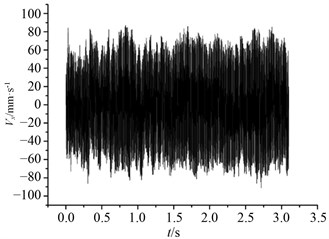a) 1 m position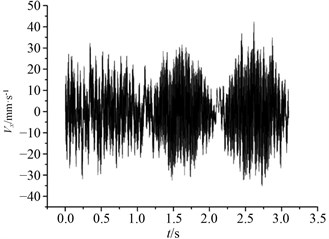b) 1.5 m position

Fig. 12Longitudinal vibration response of lifting pipe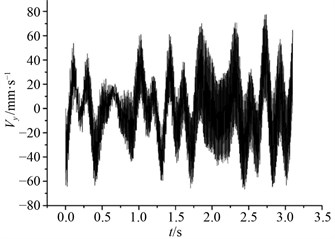a) 1 m position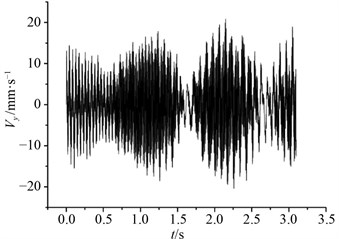b) 1.5 m position

Fig. 13Spectrum response under different water depths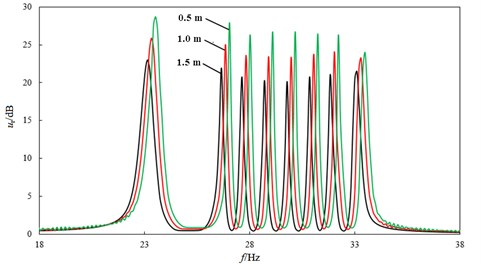The variation curve of longitudinal ($y$-direction) vibration velocity signal ${V}_{y}$ of lifting pipe model at 1 m node and 1.5 m node is shown in Fig. 12. It can be seen that under the influence of hull disturbance constraints, at the same node position, although the amplitude peak of the longitudinal vibration of the riser model is slightly smaller than that of the transverse vibration, the fluctuation is more significant. The longitudinal vibration at the 1.5 m node also shows some discontinuous linearity.

Through Fourier transform and filter processing, the spectrum response under different water depths can be obtained in Fig. 13. It can be seen that the change law of relative amplitude ${u}_{r}$ is quite consistent with the simulation results, even if the overall dimension of experimental model is different from that of lifting pipe.

## 5. Conclusions

The vibration characteristic of ship mining system is an important reference and basis to ensure the optimal design and optimal working state of the mechanism. In this paper, the nonlinear vibration characteristics of lifting system are studied by using numerical analysis and experimental test methods, and the following conclusions can be drawn:

1) The lifting pipe in shallow water bears greater load. When the water depth exceeds 1 km, the ocean current gradually decreases and tends to be stable, but the characteristic frequency of the whole lifting pipe system is very low. The influence of wind load and hull disturbance velocity on the amplitude of lifting pipe is very significant. Therefore, improving the docking stability of mining ship and reducing the amplitude of hull disturbance is one of the most effective methods to reduce the vibration of lifting pipe.

2) Under the restraint of anchor machine and anchor chain, the disturbance inertia force along the transverse direction of mining ship is small. Therefore, the transverse vibration feedback of mine lifting pipeline is mainly produced by the actual ocean current, and the vibration response in the longitudinal direction is more obviously affected by the law of hull disturbance. With the increase of water depth, the single effect of hull disturbance and ocean current is weakened, and the transient amplitude shows some discontinuity. The research on the nonlinear vibration characteristics of ship mining system can provide an important basis for the optimization of riser structure design and assembly process, so as to avoid the blindness of product research and development or function strengthening, and has good economic and social benefits.